# Maharashtra Board 12th Commerce Maths Solutions Chapter 1 Commission, Brokerage and Discount Miscellaneous Exercise 1

Balbharati Maharashtra State Board 12th Commerce Maths Digest Pdf Chapter 1 Commission, Brokerage and Discount Miscellaneous Exercise 1 Questions and Answers.

## Maharashtra State Board 12th Commerce Maths Solutions Chapter 1 Commission, Brokerage and Discount Miscellaneous Exercise 1

(I) Choose the correct alternative.

Question 1.
An agent who gives a guarantee to his principal that the party will pay the sale price of goods is called
(a) Auctioneer
(b) Del Credere Agent
(c) Factor
(d) Broker
(b) Del Credere Agent

Question 2.
An agent who is given the possession of goods to be sold is known as
(a) Factor
(b) Broker
(c) Auctioneer
(d) Del Credere Agent
(a) Factor

Question 3.
The date on which the period of the bill expires is called
(a) Legal Due Date
(b) Grace Date
(c) Nominal Due Date
(d) Date of Drawing
(c) Nominal Due DateQuestion 4.
The payment date after adding 3 days of grace period is known as
(a) The legal due date
(b) The nominal due date
(c) Days of grace
(d) Date of drawing
(a) The legal due date

Question 5.
The sum due is also called as
(a) Face value
(b) Present value
(c) Cash value
(d) True discount
(a) Face value

Question 6.
P is the abbreviation of
(a) Face value
(b) Present worth
(c) Cash value
(d) True discount
(b) Present worth

Question 7.
Banker’s gain is the simple interest on
(a) Banker’s discount
(b) Face Value
(c) Cash value
(d) True discount
(d) True discount

Question 8.
The marked price is also called as
(a) Cost price
(b) Selling price
(c) List price
(d) Invoice price
(c) List priceQuestion 9.
When only one discount is given then
(a) List price = Invoice price
(b) Invoice price = Net selling price
(c) Invoice price = Cost price
(d) Cost price = Net selling price
(b) Invoice price = Net selling price

Question 10.
The difference between the face value and present worth is called
(a) Banker’s discount
(b) True discount
(c) Banker’s gain
(d) Cash value
(b) True discount

(II) Fill in the blanks.

Question 1.
A person who draws the bill is called ____________
Drawee

Question 2.
An ____________ is an agent who sells the goods by auction.
Auctioneer

Question 3.
Trade discount is allowed on the ____________ price.
Catalogue/List

Question 4.
The banker’s discount is also called ____________.
Commercial DiscountQuestion 5.
The banker’s discount is always ____________ than the true discount.
higher

Question 6.
The diffrence between the banker’s discount and the true discount is called ____________.
bankers gain

Question 7.
The date by which the buyer is legally allowed to pay the amount is known as ____________.
legal due date

Question 8.
A ____________ is an agent who brings together the buyer and the seller.
broker

Question 9.
If buyer is allowed both trade and cash discounts, ____________ discount is fist calculated on ____________ price.

Question 10.
____________ = List price (catalogue Price) – Trade Discount.
Invoice Price

(III) State whether each of the following is True or False.

Question 1.
A broker is an agent who gives a guarantee to the seller that the buyer will pay the sale price of goods.
False

Question 2.
A cash discount is allowed on the list price.
FalseQuestion 3.
Trade discount is allowed on catalogue price.
True

Question 4.
The buyer is legally allowed 6 days grace period.
False

Question 5.
The date on which the period of the bill expires is called the nominal due date.
True

Question 6.
The difference between the banker’s discount and true discount is called sum due.
False

Question 7.
The banker’s discount is always lower than the true discount.
False

Question 8.
The banker’s discount is also called a commercial discount.
True

Question 9.
In general cash, the discount is more than trade discount.
False

Question 10.
A person can get both, trade discount and a cash discount.
True

(IV) Solve the following problems.

Question 1.
A salesman gets a commission of 6.5% on the total sales made by him and a bonus of 1% on sales over ₹ 50,000. Find his total income on a turnover of ₹ 75,000.
Solution:
Rate of commission = 6.5% on the total sales
∴ Commission on a turnover of ₹ 75,000
= $$\frac{6.5}{100}$$ × 75,000
= ₹ 4,875
Rate of bonus = 1% on sales over ₹ 50,000
∴ Amount of bonus = $$\frac{1}{100}$$ × (75,000 – 50,000) = ₹ 250
∴ Total income of the sales man = ₹ 4,875 + ₹ 250 = ₹ 5,125Question 2.
A shop is sold at 30% profit, the amount of brokerage at the rate of $$\frac{3}{4}$$% amounts to ₹ 73,125. Find the cost of the shop.
Solution:
Rate of brokerage = $$\frac{3}{4}$$%
Amount of brokerage = ₹ 73,125
Let the selling price of the shop be ₹ 100 then the brokerage = ₹ $$\frac{3}{4}$$
Thus, if the amount of brokerage is ₹ $$\frac{3}{4}$$ then the selling price of the shop is ₹ 100
If the amount of brokerage is ₹ 73,125, then the selling price of the shop is = 73125 × $$\frac{4}{3}$$ × 100 = ₹ 97,50,000
The shop is sold at 30% profit
∴ If the cost of the shop is ₹ 100, then it is sold at ₹ 130
Thus, if the shop is sold at ₹ 130, then its cost price is ₹ 100
If the shop is sold at ₹ 97,50,000 then its cost price is = $$\frac{97,50,000 \times 100}{130}$$ = ₹ 75,00,000
Then, the cost of the shop is ₹ 75,00,000

Question 3.
A merchant gives 5% commission and 1.5% delcredere to his agents. If the agent sells goods worth ₹ 30,600 how much does he get? How much does the merchant receive?
Solution:
Rate of commission = 5%
Total sales = ₹ 30,600
Amount of commission = $$\frac{5}{100}$$ × 30,600
Rate of delcredere = 1.5%
= $$\frac{1.5}{100}$$ × 30,600
= ₹ 459
Thus, the agents gets 1,530 + 459 = ₹ 1,989
And the merchant receives = 30,600 – 1,989 = ₹ 28,611

Question 4.
After deducting commission at 7$$\frac{1}{2}$$% on first ₹ 50,000 and 5% on the balance of sales made by him, an agent remits ₹ 93,750 to his principal. Find the value of goods sold by him.
Solution:
Rate of commission = 7$$\frac{1}{2}$$% on first ₹ 50,000
= $$\frac{7.5}{100}$$ × 50,000
= ₹ 3,750
Let the total sales be ₹ x
Rate of commission on the balance sales = 5%
Commission on the balance sales = $$\frac{5}{100}$$ × (x – 50000) = $$\frac{x}{20}$$ – 2,500
Total commission = 3750 + $$\frac{x}{20}$$ – 2,500 = $$\frac{x}{20}$$ + 1,250
Now, the amount to be remitted to the principal = Value of goods sold – Commission of the agent
= x – ($$\frac{x}{20}$$ + 1250)
= $$\frac{19x}{20}$$ – 1250
The agents remits ₹ 93,750 to his principal
∴ $$\frac{19x}{20}$$ – 1,250 = 93,750
∴ $$\frac{19x}{20}$$ = 95,000
∴ x = ₹ 1,00,000
Thus, the value of the goods sold by the agent is ₹ 1,00,000Question 5.
The present worth of ₹ 11,660 due 9 months hence is ₹ 11,000. Find the rate of interest.
Solution:
Given, PW = ₹ 11,000, SD = ₹ 11,660
n = $$\frac{9}{12}$$ year = $$\frac{3}{4}$$ year
We have,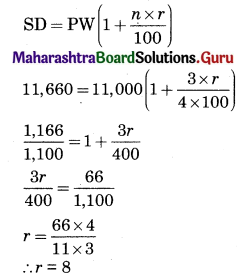∴ The rate of interest is 8% p.a.

Question 6.
An article is marked at ₹ 800, a trader allows a discount of 2.5% and gains 20% on the cost. Find the cost price of the article?
Solution:
Marked price of the article = ₹ 800
Rate of discount = 2.5%
Amount of discount = $$\frac{2.5}{100}$$ × 800 = ₹ 20
∴ Selling price of the article = 800 – 20 = ₹ 780
Now, given, gain = 20%
Let cost price of the article be ₹ 100, then
The selling price of the article is ₹ 120
Thus if cost price of the articles is ₹ x
Then the selling price is ₹ 780
∴ x = $$\frac{780 \times 100}{120}$$
∴ x = 650
∴ Cost price of the article is ₹ 650

Question 7.
A salesman is paid a fixed monthly salary plus commission on the sales. If on sale of ₹ 96,000 and ₹ 1,08,000 in two successive months he receives in all ₹ 17,600 and ₹ 18,800 respectively. Find his monthly salary and rate of commission paid to him.
Solution:
Let the monthly salary of the salesman be ₹ x
And the rate of commission be y%
Income = monthly salary + commission on the sales
17600 = x + $$\frac{y}{100}$$ × 96,000
∴ 17600 = x + 960y ………(1)
and 18800 = x + $$\frac{y}{100}$$ × 108000
∴ 18,800 = x + 1080y ………(2)
Subtracting equation (1) from equation (2), we get
1,200 = 120y
∴ y = 10
Substituting y = 10 in (1), we get
17,600 = x + 960(10)
∴ x = 17,600 – 9,600 = 8,000
∴ Salary of the salesman = ₹ 8,000
Rate of commission = 10%

Question 8.
A merchant buys some mixers at a 15% discount on catalogue price. The catalogue price is ₹ 5,500 per price of the mixer. The freight charges amount to 2$$\frac{1}{2}$$% on the catalogue price. The merchant sells each mixer at a 5% discount on the catalogue price. His net profit is ₹ 41,250, Find the number of mixers.
Solution:
Catalogue price of a mixer = ₹ 5,500
Trade discount = 15% on catalogue price
= $$\frac{15}{100}$$ × 5,500
= ₹ 825
Freight charges = 2$$\frac{1}{2}$$% of the catalogue price
= $$\frac{5}{2} \times \frac{1}{100} \times 5,500$$
= ₹ 137.5
∴ Cost price of a mixer for the merchant = 5,500 – 825 + 137.5 = 4,812,5
Catalogue price = ₹ 5,500
Rate of discount = 5%
∴ Selling price of one mixer = 5500 – $$\frac{5}{100}$$ × 5,500 = ₹ 5,225
∴ Profit on one mixer = 5,225 – 4,812.5 = ₹ 412.5
Now, total profit = ₹ 41,250
∴ Number of mixers = $$\frac{41,250}{412.5}$$ = 100
Thus the number of mixers is 100.Question 9.
A bill is drawn for ₹ 7,000 on 3rd May for 3 months and is discounted on 25th May at 5.5% Find the present worth.
Solution:
Face value of the bill = ₹ 7,000
Date of drawing = 3rd May
Period = 3 months
Normal due date = 3rd August
Legal due date = 6th August
Rate of interest = 5.5%
Date of discounting = 25th May
Unexpired period (number of days from date of discounting to legal due date)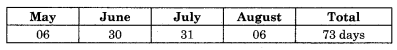∴ Bankers discount = 7,000 × $$\frac{73}{365} \times \frac{5.5}{100}$$ = ₹ 77
Also PW = SD – BD
= 7,000 – 77
= ₹ 6,923
∴ Present worth is ₹ 6,923

Question 10.
A bill was drawn on 14th April 2005 for ₹ 3,500 and was discounted on 6th July 2005 at 5% per annum. The banker paid ₹ 3,465 for the bill. Find the period of the bill.
Solution:
Face value of the bill = ₹ 3,500
Date of drawing = 14/04/2005
Date of discount = 06/07/2005
Rate of interest = 5%
Cash value = ₹ 3,465
Bankers discount = Face value – Cash value
= 3,500 – 3,465
= ₹ 35
Let the unexpired days be n days
∴ BD = $$\frac{\mathrm{FV} \times n \times r}{365 \times 100}$$
∴ 35 = $$\frac{3,500 \times n \times 5}{365 \times 100}$$
∴ n = 73 days
Thus, legal due date is 73 days from the date of discounting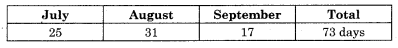∴ Legal due date = 17/09/2005
∴ Nominal due date = 14/09/2005
∴ The period of the bill is 5 months

Question 11.
The difference between true discount and banker’s discount on 6 months hence at 4% p.a. is ₹ 80. Find the true discount, banker’s discount, and amount of the bill.
Solution:
BG = BD – TD
∴ BG = ₹ 80
Also BG = $$\frac{\mathrm{TD} \times n \times r}{100}$$
∴ 80 = $$\frac{\mathrm{TD} \times 6 \times 4}{12 \times 100}$$
∴ TD = $$\frac{80 \times 100}{2}$$
∴ TD = ₹ 4,000
Now BD = TD + BG
= 4,000 + 80
= ₹ 4,080
Also, BD = $$\frac{\mathrm{FV} \times n \times r}{100}$$
∴ 4,080 = $$\frac{\mathrm{FV} \times 6 \times 4}{12 \times 100}$$
∴ FV = $$\frac{4,080 \times 100}{2}$$
∴ FV = ₹ 2,04,000
Amount of the bill = ₹ 2,04,000Question 12.
A manufacturer makes a clear profit of 30% on the cost after allowing a 35% discount. If the cost of production rises by 20%, by what percentage should he reduce the rate of discount so as to make the same rate of profit keeping his list prices unaltered.
Solution:
Rate of discount = 35%
Let the list price be ₹ 100.
Then discount at 35% = ₹ 35
∴ Net selling price = 100 – 35 = ₹ 65 ……..(1)
The manufacturer makes a clear profit of 30% on the cost after allowing a 35% Discount.
Let the cost be ₹ 100.
Then selling price at 30% profit is 100 + 30 = ₹ 130.
Thus, if the net selling price is ₹ 130, then the cost price is ₹ 100.
But, the net selling price is ₹ 130, then the cost price is ₹ 65 ……[from (1)]
∴ The cost price is $$\frac{65}{130} \times 100$$ = ₹ 50
Hence, we have,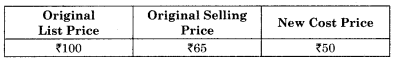Now, the cost of production has increased by 20%.
Let the old cost price be ₹ 100.
∴ The new cost price is ₹ 120.
But, the old cost price is ₹ 50.
∴ The new cost price is = $$\frac{50}{100} \times 120$$ = ₹ 60.
The old net price is ₹ 65.
Now 20% of ₹ 65 = $$\frac{20}{100} \times 65$$ = ₹ 13
∴ New net price = 65 + 13 = ₹ 78
Hence, we have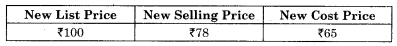Now, 100 – 78 = ₹ 22
Thus, the rate of discount should be reduced by 22%, The original rate of discount is 35%.
Hence, the reduction in discount should be (35 – 22)% = 13%
so as to make the same rate of profit, keeping the list price unaltered.

Question 13.
A trader offers a 25% discount on the catalogue price of the radio and yet makes a 20% profit. If he gains ₹ 160 per radio, what must be the catalogue price of the radio?
Solution:
Rate of discount = 25% on the catalogue price of a radio.
Let the catalogue price of the radio be ₹ 100.
Then, the discount on a radio = ₹ 25.
Net selling price = 100 – 25 = ₹ 75.
He makes a profit of 20%.
Let the cost price be ₹ 100.
Then, at 20% profit, net selling price = ₹ 120.
Thus, if net SP is ₹ 120, then cost price is ₹ 100.
But, the net SP is ₹ 75.
∴ The cost price is $$\frac{75}{120}$$ × 100 = $$\frac{750}{12}$$ = ₹ 62.50
∴ Profit on a radio set = 75 – 62.5 = ₹ 12.50
Thus, if the profit on a radio set is ₹ 12.50 then its catalogue price is ₹ 100.
But the profit on a radio set is ₹ 160.
∴ The catalogue price of radio = $$\frac{160}{12.50}$$ × 100
= 12.80 × 100
= ₹ 1,280
∴ Thus, the catalogue price of the radio is ₹ 1280

Question 14.
A bill of ₹ 4,800 was drawn on 9th March 2006 at 6 months and was discounted on 19th April 2006 for 6$$\frac{1}{4}$$% p.a. How much does the banker charge and how much does the holder receive?
Solution:
Face value of the bill = ₹ 4.800
Date of drawing = 09/03/2006
Period of the bill = 6 months
Normal due date = 09/09/2006
Legal due date = 12/09/2006
Rate of discount = 6$$\frac{1}{4}$$% = 6.25%
Now, for the unexpired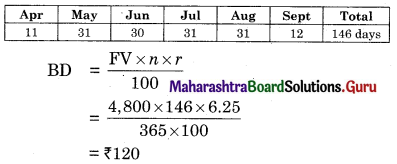Thus the banker charges ₹ 120
Amount received by the holder = 4,800 – 120 = ₹ 4,680

Question 15.
A bill of ₹ 65,700 drawn on July 10 for 6 months was discounted for ₹ 65,160 at 5% p.a. On what day was the bill discounted?
Solution:
BD = FV – Cash value
= 65,700 – 65,160
= ₹ 540
Let the unexpired days be x days
BD = $$\frac{\mathrm{FV} \times n \times r}{100}$$
∴ 540 = $$\frac{65,700 \times x \times 5}{365 \times 100}$$
∴ x = 60 days
The unexpired days = 60 days
Date-of drawing = 10th July
Period of the bill = 6 months
Nominal due date = 10th January (next year)
Legal due date = 13th January (next year)
Then the date of discount is 60 days before, the legal due date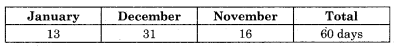∴ The date of discounting is 14th November

Question 16.
An agent sold a car and charged a 3% commission on the sale value. If the owner of the car received ₹ 48,500, find the sale value of the car. If the agent charged 2% from the buyer, find his total remuneration.
Solution:
Let the sale value of the car be ₹ x
Rate of commission of the agent = 3%
Since the owner received ₹ 48,500 after agent has charged his commission
x – $$\frac{3 x}{100}$$ = 48500
∴ $$\frac{97 x}{100}$$ = 48500
∴ x = $$\frac{48,500 \times 100}{97}$$
∴ x = ₹ 50,000
∴ Sale value of the car = ₹ 50,000
Against commission received from the owner = $$\frac{3}{100}$$ × 50,000 = ₹ 1500
Against commission received from the buyer = $$\frac{2}{100}$$ × 50,000 = ₹ 1000
∴ Agents total remuneration = 1,500 + 1,000 = ₹ 2,500Question 17.
An agent is paid a commission of 4% on cash sales and 6% on credit sales made by him. If on the sale of ₹ 51,000 the agent claims a total commission of ₹ 2,700, find the sales made by him for cash and on credit.
Solution:
Total sales = ₹ 51,000
Let eash sales be ₹ x
∴ Credit sales = ₹ (51,000 – x)
Agent’s commission on cash sales = 4%
= $$\frac{4}{100}$$ × x
= $$\frac{4x}{100}$$
Commission on credit sales = 6%
= $$\frac{6}{100}$$(51,000 – x)
Given total commission = ₹ 2,700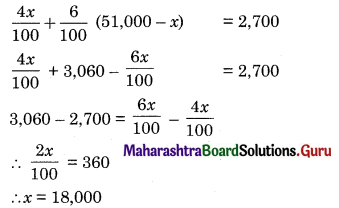∴ Cash sales = ₹ 18,000
∴ Credit sales = 51,000 – 18,000 = ₹ 33,000